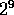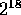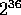Next: Future Improvements Up: Real-Time Ray Tracing on Previous: The Algorithm

# The Implementation

The biggest challenge with this implementation was the limited memory on the renderers---208 bits per processor. This limit, along with the desire to have at least a 1280x1024 display (and thus allow object positions anywhere in this range), limited the implementation of the algorithm to integer arithmetic; fixed-point would have taken too many additional bits. Fixed-point is used at one point, as noted below, but the ray parametric parameters, the model description, and most intermediate values are kept as 12 or 24 bit integers.

With most integers being on the order of 500, or, problems arose when calculating parts of the intersection formula. Namely, the coefficients of the quadratic equation were on the order of the squares of the integers, or, and they themselves are squared in the determinant of the quadratic formula, to yield numbers on the order of. The square root function needs an equal number of bit for the input, the output, and some temporary space, so well over half the the memory needs to be free just for the square root operation!

This problem led to the use of the Backing Store. The ray, which is kept in six 12-bit integers, is swapped out to the Backing Store after the coefficients of the quadratic equation are calculated. It stays there until the parametric value T is calculated, whereupon it is swapped back in and the intersection point is found. The Backing Store is used a few other times to save important values while their space is used for temporary variables.

To increase interactivity, the scene is rendered at one-quarter the resolution (640x512) while the joystick is active; when the joystick stops, the full resolution (1280x1024) is rendered.

Although the original idea was to use fixed-point for all variables, some problems arose with the calculation of the parametric value T. When the normal of the sphere is calculated, 12 bits are used to store each of the normal's components, and the normal is simply kept as the length of the sphere's radius. This yields a normal which has length on the order of 200. When such a ray is intersected with another sphere which is on the order of 400 units away, the parametric value T truncates to 0, 1, or 2. To fix this, an additional 6 bits were added to the right of T's binary point, which significantly helped the accuracy of the reflections. This bias is removed after the value is plugged back into the ray.Next: Future Improvements Up: Real-Time Ray Tracing on Previous: The Algorithm

Lawrence Kesteloot
Tue Jan 24 16:36:27 EST 1995